Next: Subroutine Package for Nonlinear Up: Incoherent Pair Production Previous: Breit-Wheeler Process

### Virtual (almost real) photon approximation

To treat the Bethe-Heitler , Landau-Lifshitz  and the Bremsstrahlung processes, the so-called almost-real-photon approximation, or equivalent photon approximation, or Weizacker-Williams approximation, is employed. An electron is accompanied by virtual photons which look like real photons at ultra-relativistic limit. They interact with on-coming (real or virtual) photons incoherently. Thus, the Bethe-Heitler and Landau-Lifshitz processes above are reduced to the Breit-Wheeler process and the Bremsstrahlung to the Compton process:

Bethe-Heitler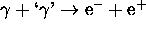Landau-Lifshitz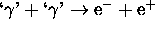Bremsstrahlung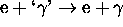where `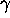' is a virtual photon.

Let the electron energy be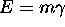(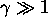). The number of virtual photons with energy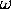and transverse momentum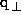is given by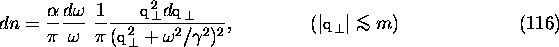where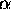is the fine structure constant. For given, the typical transverse momentum is very small,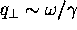, so that it is not important in collision kinematics but, instead, the finite transverse extent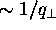can bring about significant effects. In the (transverse) configuration space, the above expression becomes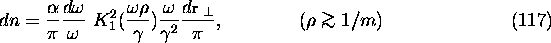where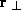is the transverse coordinate with respect to the parent electron,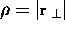, and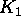the modified Bessel function.

The transverse momentum cut off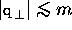(or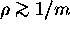) is somewhat umbiguous. It should depend on the momentum transfer of the whole process. This dependence is ignored in CAIN because the virtual photons are generated independently from the following processes and because it does not much affect the low energy pairs.

The lower limit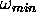of the integration overis, in our case, determined by the pair creation threshold. Let us introduce dimensionless variables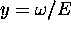,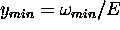, and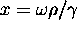. The total number of the virtual photons is given by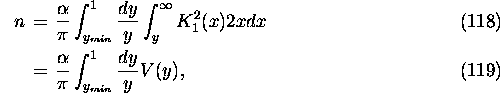with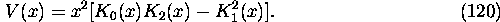When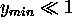, the total number is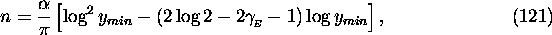where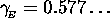is Euler's constant. At very high energies the number of virtual photons per electron is O(1), in spite of the small factor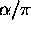, due to the factor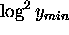.

1ex

When Bethe-Heitler and/or Landau-Lifshitz processes are specified by PPINT command, CAIN generates virtual photons in each longitudinal slice at each time step and counts them in the same mesh as that generated by the LUMINOSITY command. The number of macro-virtual photons is somewhat arbitrary. In the present version it is determined such that the weight of the macro-virtual photons is equal to the maximum weight of the electrons in the on-coming beam (not equal to the weight of each parent electron in order to prevent low-weight electrons from generating many photons).

Since the y (energy) spectrum is approximately proportional to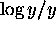for small y, the spectrum becomes almost flat if one chooses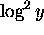as the primary variable. To account for relatively large y too, CAIN adopts the variable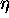instead of y: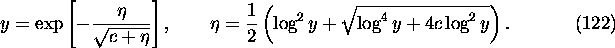Here, c>0 is introduced so that the function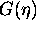defined later, is finite. It is chosen to be 0.2 but is almost arbitrary provided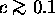. The maximumis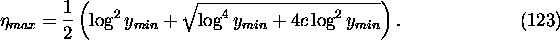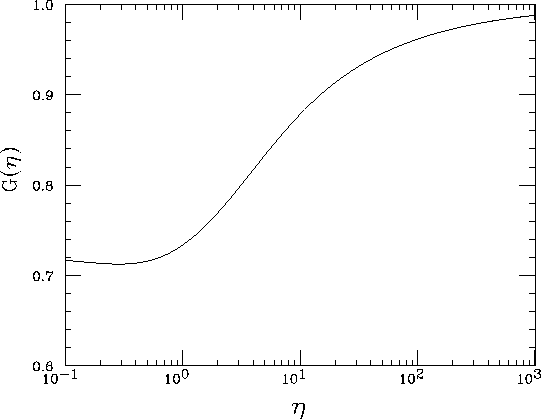Figure 11: Functiondefined in eq.(125). It is close to unity because only largeregion is important. G(0) is finite and depends on the parameter c. G(0)<1 if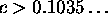. Here, c=0.2 is adopted.

Now, the spectrum with respect tois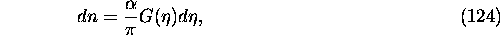with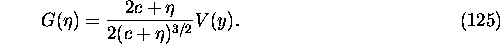For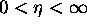,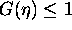and close to 1 except for the smallregion which is umimportant in practice. Thus,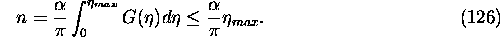For given(or y) the distribution of x is proportional to dV(x)/V(y) and, therefore, can be random-generated by using inverse function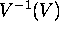.

The algorithm is as follows.

(a)
From the given parameters, compute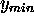,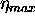and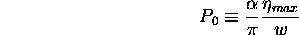where w is the weight of virtual photon to be created.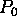is the expected number of macro-virtual photons. Ifis not small enough (say, >0.1), divide it by an integer N and repeat the following steps N times.
(b)
Generate a uniform random number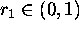. Reject if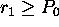. Otherwise redefine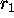by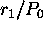.
(c)
Generate a random number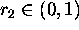, define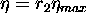and calculatefrom a table. Reject if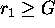. (The probability to be rejected here is small because G is close to unity.) Otherwise, accept.
(d)
Calculate y using eq.(122) and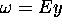. If LOCAL option is specified, stop here and return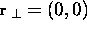. Otherwise, calculate the value of V(y) from G using eq.(125).
(e)
Generate a random number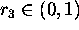and solve the equation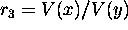with respect to x. This is done by using a table of inverse function of V.
(f)
Compute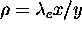,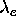being the Compton wave length.
(g)
Generate a random number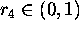and compute the photon coordinate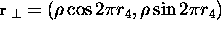.Next: Subroutine Package for Nonlinear Up: Incoherent Pair Production Previous: Breit-Wheeler Process

Toshiaki Tauchi
Thu Dec 3 17:27:26 JST 1998